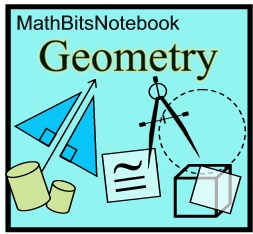Geometry section of MathBitsNotebook.comSimilarity* CCSS and PARCC specifically mention AA in relation to similar triangles. Engage NY also mentions SSS and SAS methods.
Engage NY specifies dilations not centered at the origin.
Dilations (properties, definition, scale factors, use on coordinate axes)
 Stretches (vertical and horizontal dilations) not specified in CCSS/PARCC
Similar Figures (definitions, similarity transformations)
 Proving Similar Triangles (AA (proof), SSS, SAS) see * above
"Side Splitter" Theorem (theorem, converse, proofs, example)
 (theorem, proof, example) not specified in CCSS/PARCC
Measurements in Similar Figures (perimeters, areas, volumes)
Proof Problems for Similar Triangles (similar triangles, proportions, products)
Construct: Similar Triangles (Note: this links to Construction section.)

Similarity in relation to Circles Proofs appears under Circles

 PRACTICE: • Practice with Dilations • Practice with Dilations and Lines • Practice with Similarity (numerical problems) • Practice with Similarity Measurements • Practice with Side Splitter Theorem • Practice with Angle Bisector Theorem • Practice with Similarity Proofs

 REFRESHERS: (while these topics are mostly "review", you may also find new information here) * • Transformations - Dilations (intuitive, similarity, on coordinate axes) Note: this links to the Transformation section. • Ratios and Proportions (rules, properties)